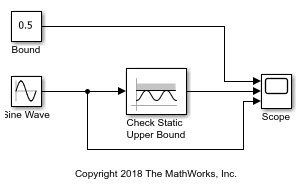# Check Signal Upper Bound with Check Static Upper Bound Block

Using the Check Static Upper Bound block, you can check if an input signal exceeds a defined value.In this example, the Check Static Upper Bound block compares the value of a Sine Wave block to the value entered in the Upper bound parameter, which is `0.5`. The Constant block, labeled Bound, illustrates this parameter in the Scope block but does not affect the parameter value. The Check Static Upper Bound block checks if the value of the input signal is less than the Upper bound. If it is, the block asserts `true (1)`. Because the Output assertion signal parameter of the block is selected, the block outputs the assertion value. Run the simulation to observe the model output.At the start of the simulation, the Check Static Upper Bound block outputs `1` because the value of the Sine Wave block is less than the bound. At a time of `0.52`, the sine wave exceeds `0.5`. The Check Static Upper Bound block recognizes this change and outputs `0`. When the time reaches `2.62`, the sine wave falls below the bound and the assertion is `true`. This pattern repeats until the simulation end time.